# SSC JE Electrical Important MCQ Part 30

SSC JE Electrical Important MCQ Part 30

1.Determine the value of the voltage measured by the meter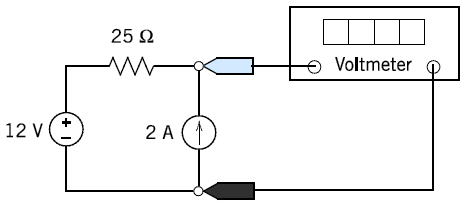A.-50 V

B. 50 V

C. 62 V

D. 64 V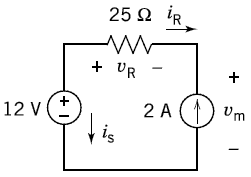vR=25 iR=25(-2)=-50 V

vm=12-vR=12-(-50)=62 V

2.Determine the current, i, at t = 4 s for the circuit of Figure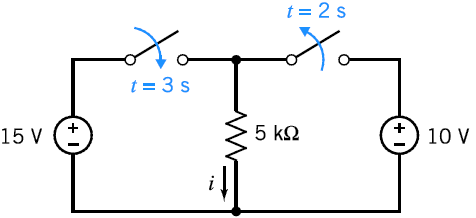A. 2mA

B. 20mA

C. 3mA

D. 30mA

At t = 4 s the left switch is closed and the right switch is open so the voltage across the resistor is 15 V.

i=V/R=15/5×10³=3 mA

3.The voltmeters in the circuit shown in Figure indicate that va = 2 V and vb = 8 V. Determine the value of b, the gain of the VCVS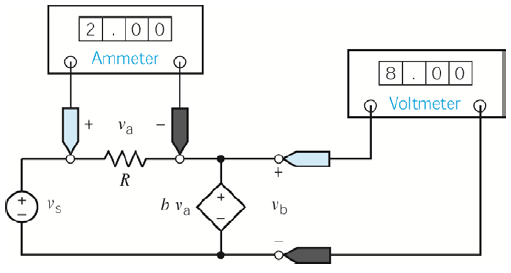A. 6 V

B. 8 V

C. 10 V

D. 4 V

va=2 V

bva=vb=8 V

So b=vb/va=8/2=4 V

4.The current source in Figure supplies 40 W. What values do the meters in Figure readA. i = -2 A,v=20 V

B. i = -4 A,v=40 V

C. i = -1 A,v=10 V

D. i = -0 A,v=5 V

The voltmeter current is zero so the ammeter current is equal to the current source current except for the reference direction i = -2 A

The voltage v is the voltage of the current source. The power supplied by the current source is 40 W so

40=2v OR v=40/2=20 V

5.In the circuit below, the battery has negligible internal resistance. Lamps L, M and N which have different resistance are connected as shown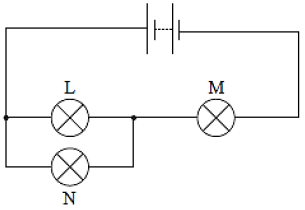Which one of the following is always true

A. Lamps L and N have the same current through them

B. Lamps L and M have the same current through them

C. Lamps L and N have the same potential difference across them

D. Lamps L and M have the same potential difference across them

Answer : C (Lamps L and N have the same potential difference across them because voltage always same in parallel branch)

Electrical One Year Test Series Pass-Daily Test Plus Weekly Full Test

6.The current flowing in the main circuit is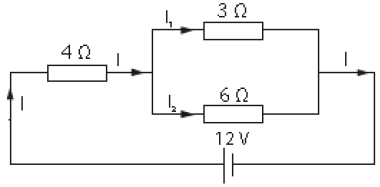A. 2 A

B. 4 A

C. 6 A

D. 3 A

Parallel circuit 1 / Reff = 1 / R1 + 1 / R2 = 1/3 + 1/6 = 1/2 OR Reff = 2 Ω

Total resistance: Reff = 4 Ω + 2 Ω = 6 Ω

I = V/R = 12/6 = 2 A

7.Determine the current IX in the following circuit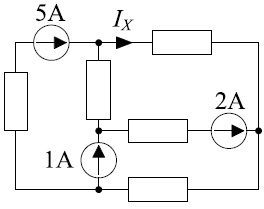A. 5 A

B. -5 A

C. 4 A

D. -4 A

IX-5-1+2 =0 Hence IX = 4 A

8.Determine the voltage VX in the following circuitA. 8V

B. -8V

C. 9V

D. -9V

VX = (-3) + (+2) + (+9) = +8V

9.Two 1.0 kΩ resistors are in series and this series combination is in parallel with a 2.2 kΩ resistor. The voltage across one of the resistors is 6 V. The voltage across the 2.2 kΩ resistor is

A. 6 V

B. 3 V

C. 12 V

D. 13.2 V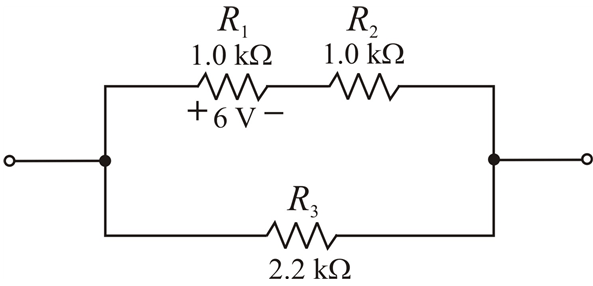Voltage across series resistance will be same because series resistance is same so voltage=6+6=12 V

Voltage across parallel will be same,so total voltage across 2.2 kΩ resistor =12 V

Electrical One Year Test Series Pass-Daily Test Plus Weekly Full Test

10.The total resistance of Figure can be found with which of the following formula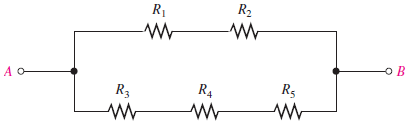A. R1 + R2 + R3 ⁄⁄ R4 ⁄⁄ R5

B. R1 ⁄⁄ R2 + R3 ⁄⁄ R4 ⁄⁄ R5

C. (R1 + R2) ⁄⁄ (R3 + R4 + R5)

D. none of these

The R1 & R2 in series =(R1 + R2)

Again R3,R4 & R5 in series=(R3 + R4 + R5)

Both these are in parallel so equivalent resistance will be

(R1 + R2) ⁄⁄ (R3 + R4 + R5)

11.The advantage of salient poles in an alternator is

A. Reduced noise

B. Reduced windage loss

C. Reduced bearing loads and noise

D. Adaptability to low and medium speed operation

Answer : D (Salient pole generators will have typical speed about 100 to 375 rpm/low and medium speed)

12.The operating time of the back-up relay should be more than

A. The primary relay time

B. The primary fault clearing time

C. The critical clearing time

D. The pick up time

Answer : B (The operating time of the back-up relay should be more than the primary fault clearing time)

13.Equalizing circuits are provided across each SCR in series operation to provide uniform

A. Current distribution

B. Voltage distribution

C. Firing of SCR’s

D. dv/dt protection

14.Which of the following is false? Skin effect is found to increase with increase in

A. Conductor cross section

B. Supply frequency

C. Voltage

D. Permeability

Answer : C (Factors affecting skin effect=Frequency ,Diameter,The shape of the conductor,Type of material)

15.In the star-delta starter of an induction motor

A. Applied voltage per stator phase is 67.7% of the line voltage

B. Applied voltage per stator phase is 37.4% of the line voltage

C. Applied voltage per stator phase is 70.7% of the line voltage

D. Applied voltage per stator phase is 57.7% of the line voltage

Electrical One Year Test Series Pass-Daily Test Plus Weekly Full Test

16.When a d.c. generator, is operating at constant speed, variable load, which loss is likely to vary significantly

A. Windage loss

B. Eddy current loss

C. Bearing friction loss

D. Copper loss

17.Which device is used for the measurement of low pressure below the atmospheric pressure

A. Pirani guage

B. Compound gauge

C. Strain guage

D. Ionization guage

18.In a transistor, the conductivity of the

A. Emitter region is lower than the base region

B. Emitter and base regions is of the same order

C. Emitter region is higher than the base region

D. Emitter and base regions is zero

19.The desirable qualities of a power system are

A. High load factor and high diversity factor

B. Low load factor and low diversity factor

C. Low load factor and high diversity factor

D. High load factor and low diversity factor

20.Field Effect Transistor (FET) is a device having

A. High input impedance and is voltage controlled

B. Low input impedance and is voltage controlled

C. High input impedance and is current controlled

D. Low input impedance and is current controlled